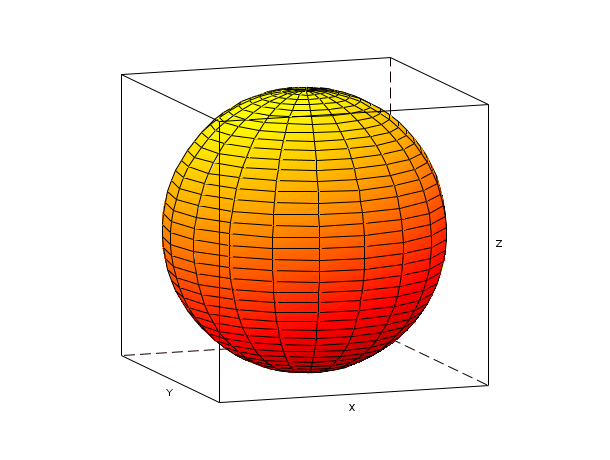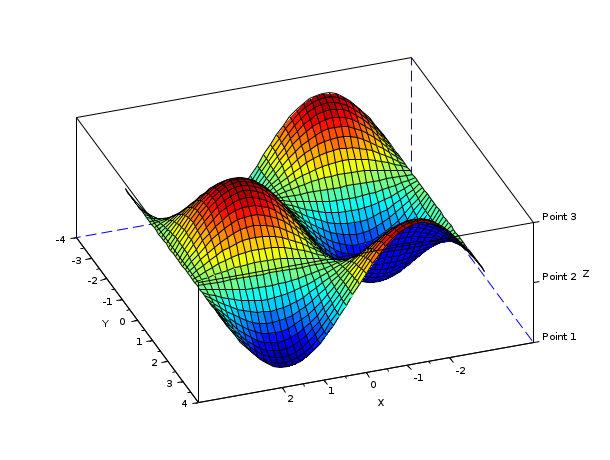Scilab Home page | Wiki | Bug tracker | Forge | Mailing list archives | ATOMS | File exchange
Change language to: English - Français - Português - 日本語 -

See the recommended documentation of this function

Справка Scilab >> Графики > 3d_plot > plot3d

# plot3d

3D plot of a surface

### Syntax

```plot3d(x,y,z,[theta,alpha,leg,flag,ebox])
plot3d(x,y,z,<opt_args>)

plot3d(xf,yf,zf,[theta,alpha,leg,flag,ebox])
plot3d(xf,yf,zf,<opt_args>)

plot3d(xf,yf,list(zf,colors),[theta,alpha,leg,flag,ebox])
plot3d(xf,yf,list(zf,colors),<opt_args>)

plot3d(z)```

### Arguments

x,y

row vectors of sizes n1 and n2 (x-axis and y-axis coordinates). These coordinates must be monotone.

z

matrix of size (n1,n2). `z(i,j)` is the value of the surface at the point (x(i),y(j)).

xf,yf,zf

matrices of size (nf,n). They define the facets used to draw the surface. There are `n` facets. Each facet `i` is defined by a polygon with `nf` points. The x-axis, y-axis and z-axis coordinates of the points of the ith facet are given respectively by `xf(:,i)`, `yf(:,i)` and `zf(:,i)`.

colors

a vector of size n giving the color of each facets or a matrix of size (nf,n) giving color near each facet boundary (facet color is interpolated ).

<opt_args>

This represents a sequence of statements ```key1=value1, key2=value2``` ,... where `key1`, `key2,...` can be one of the following: theta, alpha ,leg,flag,ebox (see definition below).

theta, alpha

real values giving in degree the spherical coordinates of the observation point (by default, `alpha`=35° and `theta`=45°).

leg

string defining the labels for each axis with @ as a field separator, for example "X@Y@Z" (by default, axis have no label).

flag

a real vector of size three. `flag=[mode,type,box]`(by default `flag`=[2,8,4]).

mode

an integer (surface color).

mode>0

the surface is painted with color `"mode"` ; the boundary of the facet is drawn with current line style and color.

mode=0:

a mesh of the surface is drawn.

mode<0:

the surface is painted with color `"-mode"` ; the boundary of the facet is not drawn.

Note that the surface color treatment can be done using `color_mode` and `color_flag` options through the surface entity properties (see surface_properties).

type

an integer (scaling).

type=0:

the plot is made using the current 3D scaling (set by a previous call to `param3d`, `plot3d`, `contour` or `plot3d1`).

type=1:

rescales automatically 3d boxes with extreme aspect ratios, the boundaries are specified by the value of the optional argument `ebox`.

type=2:

rescales automatically 3d boxes with extreme aspect ratios, the boundaries are computed using the given data.

type=3:

3d isometric with box bounds given by optional `ebox`, similarily to `type=1`.

type=4:

3d isometric bounds derived from the data, to similarily`type=2`.

type=5:

3d expanded isometric bounds with box bounds given by optional `ebox`, similarily to `type=1`.

type=6:

3d expanded isometric bounds derived from the data, similarily to `type=2`.

Note that axes boundaries can be customized through the axes entity properties (see axes_properties).

box

an integer (frame around the plot).

box=0:

nothing is drawn around the plot.

box=1:

unimplemented (like box=0).

box=2:

only the axes behind the surface are drawn.

box=3:

a box surrounding the surface is drawn and captions are added.

box=4:

a box surrounding the surface is drawn, captions and axes are added.

Note that axes aspect can also be customized through the axes entity properties (see axes_properties).

ebox

It specifies the boundaries of the plot as the vector `[xmin,xmax,ymin,ymax,zmin,zmax]`. This argument is used together with `type` in `flag` : if it is set to `1`, `3` or `5` (see above to see the corresponding behaviour). If `flag` is missing, `ebox` is not taken into account (by default `ebox` is missing).

Note that, when specified, the `ebox` argument acts on the `data_bounds` field that can also be reset through the axes entity properties (see axes_properties).

### Description

`plot3d(z)` draws the parametric surface `z=f(x,y)` where `x=1:m`, `y=1:n` and `[m,n]=size(z)` where `m` and `n` must be greater than 1.

`plot3d(x,y,z,[theta,alpha,leg,flag,ebox])` draws the parametric surface `z=f(x,y)`.

`plot3d(xf,yf,zf,[theta,alpha,leg ,flag,ebox])` draws a surface defined by a set of facets. You can draw multiple plots by replacing `xf`, `yf` and `zf` by multiple matrices assembled by rows as `[xf1 xf2 ...]`, `[yf1 yf2 ...]` and `[zf1 zf2 ...]`. Note that data can also be set or get through the surface entity properties (see surface_properties).

You can give a specific color for each facet by using `list(zf,colors)` instead of `zf`, where `colors` is a vector of size `n`. If `colors(i)` is positive it gives the color of facet `i` and the boundary of the facet is drawn with current line style and color. If `colors(i)` is negative, color id `-colors(i)` is used and the boundary of the facet is not drawn.

It is also possible to get interpolated color for facets. For that the color argument must be a matrix of size nfxn giving the color near each boundary of each facets. In this case positive values for colors mean that the boundary are not drawn. Note that colors can also be set through the surface entity properties (via `tlist` affectations) and edited using `color_flag` option (see surface_properties).

The optional arguments ```theta, alpha, leg ,flag, ebox``` ,can be passed by a sequence of statements `key1=value1, key2=value2`, ... In this case, the order has no special meaning. Note that all these optional arguments except `flag` can be customized through the axes entity properties (see axes_properties). As described before, the `flag` option deals with surface entity properties for `mode` (see surface_properties) and axes properties for `type` and `box` (see axes_properties).

You can use the function `genfac3d` to compute four sided facets from the surface `z=f(x,y)`. `eval3dp` can also be used.

Enter the command `plot3d()` to see a demo.

### Examples

```// simple plot using z=f(x,y)
t=[0:0.3:2*%pi]';
z=sin(t)*cos(t');
plot3d(t,t,z)``````t=[0:0.3:2*%pi]';
z=sin(t)*cos(t');
// same plot using facets computed by genfac3d
[xx,yy,zz]=genfac3d(t,t,z);
plot3d(xx,yy,zz)``````// multiple plots
t=[0:0.3:2*%pi]';
z=sin(t)*cos(t');
// same plot using facets computed by genfac3d
[xx,yy,zz]=genfac3d(t,t,z);
plot3d([xx xx],[yy yy],[zz 4+zz])``````// multiple plots using colors
t=[0:0.3:2*%pi]';
z=sin(t)*cos(t');
// same plot using facets computed by genfac3d
[xx,yy,zz]=genfac3d(t,t,z);
plot3d([xx xx],[yy yy],list([zz zz+4],[4*ones(1,400) 5*ones(1,400)]))``````// simple plot with viewpoint and captions
plot3d(1:10,1:20,10*rand(10,20),alpha=35,theta=45,flag=[2,2,3])``````// plot of a sphere using facets computed by eval3dp
deff("[x,y,z]=sph(alp,tet)",["x=r*cos(alp).*cos(tet)+orig(1)*ones(tet)";..
"y=r*cos(alp).*sin(tet)+orig(2)*ones(tet)";..
"z=r*sin(alp)+orig(3)*ones(tet)"]);
r=1; orig=[0 0 0];
[xx,yy,zz]=eval3dp(sph,linspace(-%pi/2,%pi/2,40),linspace(0,%pi*2,20));
clf();plot3d(xx,yy,zz)``````f=gcf();
f.color_map = hotcolormap(128);
r=0.3;orig=[1.5 0 0];
deff("[x,y,z]=sph(alp,tet)",["x=r*cos(alp).*cos(tet)+orig(1)*ones(tet)";..
"y=r*cos(alp).*sin(tet)+orig(2)*ones(tet)";..
"z=r*sin(alp)+orig(3)*ones(tet)"]);
[xx,yy,zz]=eval3dp(sph,linspace(-%pi/2,%pi/2,40),linspace(0,%pi*2,20));
[xx1,yy1,zz1]=eval3dp(sph,linspace(-%pi/2,%pi/2,40),linspace(0,%pi*2,20));
cc=(xx+zz+2)*32;cc1=(xx1-orig(1)+zz1/r+2)*32;
clf();plot3d1([xx xx1],[yy yy1],list([zz,zz1],[cc cc1]),theta=70,alpha=80,flag=[5,6,3])``````t=[0:0.3:2*%pi]'; z=sin(t)*cos(t');
[xx,yy,zz]=genfac3d(t,t,z);
plot3d([xx xx],[yy yy],list([zz zz+4],[4*ones(1,400) 5*ones(1,400)]))
e=gce();
f=e.data;
TL = tlist(["3d" "x" "y" "z" "color"],f.x,f.y,f.z,6*rand(f.z)); // random color matrix
e.data = TL;
TL2 = tlist(["3d" "x" "y" "z" "color"],f.x,f.y,f.z,4*rand(1,800)); // random color vector
e.data = TL2;
TL3 = tlist(["3d" "x" "y" "z" "color"],f.x,f.y,f.z,[20*ones(1,400) 6*ones(1,400)]);
e.data = TL3;
TL4 = tlist(["3d" "x" "y" "z"],f.x,f.y,f.z); // no color
e.data = TL4;
e.color_flag=1 // color index proportional to altitude (z coord.)
e.color_flag=2; // back to default mode
e.color_flag= 3; // interpolated shading mode (based on blue default color)
clf()
plot3d([xx xx],[yy yy],list([zz zz+4],[4*ones(1,400) 5*ones(1,400)]))
h=gce(); //get handle on current entity (here the surface)
a=gca(); //get current axes
a.rotation_angles=[40,70];
a.grid=[1 1 1]; //make grids
a.data_bounds=[-6,0,-1;6,6,5];
a.axes_visible="off"; //axes are hidden
a.axes_bounds=[.2 0 1 1];
h.color_flag=1; //color according to z
h.color_mode=-2;  //remove the facets boundary by setting color_mode to white color
h.color_flag=2; //color according to given colors
h.color_mode = -1; // put the facets boundary back by setting color_mode to black color
f=gcf();//get the handle of the parent figure
f.color_map=hotcolormap(512);
c=[1:400,1:400];
TL.color = [c;c+1;c+2;c+3];
h.data = TL;We can use the plot3d function to plot a set of patches (triangular, quadrangular, etc).

```// The plot3d function to draw patches:
// patch(x,y,[z])
// patch(x,y,[list(z,c)])
// The size of x : number of points in the patches x number of patches
// y and z have the same sizes as x
// c:
// - a vector of size number of patches: the color of the patches
// - a matrix of size number of points in the patches x number of
//   patches: the color of each points of each patches

// Example 1: a set of triangular patches

x = [0 0;
0 1;
1 1];

y = [1 1;
2 2;
2 1];

z = [1 1;
1 1;
1 1];

tcolor = [2 3]';

subplot(2,2,1);
plot3d(x,y,list(z,tcolor));
xtitle('A triangle set of patches');
// Example 2: a mixture of triangular and quadrangular patches

10,0;
15,5;
10,5];

20,10;
15,15;
10,5];

xtri = [ 0,10,10, 5, 0;
10,20,20, 5, 0;
20,20,15,10,10];

ytri = [ 0,10,20, 5,10;
10,20,20,15,20;
0, 0,15,10,20];

ztri = zeros(3,5);

subplot(2,2,3);
plot3d(xtri,ytri,ztri);
xtitle('Mixing triangle and quadrangle set of patches');

// Example 3: some rabbits

rabxtri = [ 5,  5, 2.5,  7.5, 10;
5, 15, 5,   10,   10;
15, 15, 5,   10,   15];

rabytri = [10, 10, 9.5,  2.5, 0;
20, 10, 12,   5,   5;
10   0   7    0    0];
rabztri = [0,0,0,0,0;
0,0,0,0,0;
0,0,0,0,0];

rabtricolor_byface = [2 2 2 2 2];

rabtricolor = [2,2,2,2,2;
3,3,3,3,3;
4,4,4,4,4];

0, 6;
5,11;
5, 6];

23,28;
23,28;
18,23];

1,1;
1,1;
1,1];

3,3;
4,4;
5,5];

subplot(2,2,2);
plot3d(rabxtri, rabytri, list(rabztri,rabtricolor));
h = gcf();
h.children(1).background = 1;
xtitle('A psychedelic rabbit set of patches');

subplot(2,2,4);
plot3d(rabxtri, rabytri, list(rabztri,rabtricolor_byface));
h = gcf();
h.children(1).background = 1;
xtitle('A standard rabbit set of patches');```We can also use the plot3d function to plot a set of patches using vertex and faces.

```// Vertex / Faces example: 3D example

// The vertex list contains the list of unique points composing each patch
// The points common to 2 patches are not repeated in the vertex list

vertex = [0 1 1;
0 2 2;
1 2 3;
1 1 4];

// The face list indicates which points are composing the patch.
face = [1 2 3;
1 3 4];

tcolor = [2 3]';

// The formula used to translate the vertex / face representation into x, y, z lists

xvf = matrix(vertex(face,1),size(face,1),length(vertex(face,1))/size(face,1))';
yvf = matrix(vertex(face,2),size(face,1),length(vertex(face,1))/size(face,1))';
zvf = matrix(vertex(face,3),size(face,1),length(vertex(face,1))/size(face,1))';

scf();
subplot(2,1,1);
plot3d(xvf,yvf,list(zvf,tcolor));
xtitle('A triangle set of patches - vertex / face mode - 3d');

// 2D test
// We use the 3D representation with a 0 Z values and then switch to 2D representation

// Vertex / Faces example: 3D example

// The vertex list contains the list of unique points composing each patch
// The points common to 2 patches are not repeated in the vertex list

vertex = [0 1;
0 2;
1 2;
1 1];

// The face list indicates which points are composing the patch.
face = [1 2 3;
1 3 4];

// The formula used to translate the vertex / face representation into x, y, z lists

xvf = matrix(vertex(face,1),size(face,1),length(vertex(face,1))/size(face,1))';
yvf = matrix(vertex(face,2),size(face,1),length(vertex(face,1))/size(face,1))';
zvf = matrix(zeros(vertex(face,2)),size(face,1),length(vertex(face,1))/size(face,1))';
tcolor = [2 3]';

subplot(2,1,2);
plot3d(xvf,yvf,list(zvf,tcolor));
xtitle('A triangle set of patches - vertex / face mode - 2D');
a = gca();
a.view = '2d';```How to set manually some ticks

```plot3d();
h = gca();
h.x_ticks = tlist(['ticks','locations','labels'],[-2,-1,0,1,2],['-2','-1','0','1','2']);
h.y_ticks = tlist(['ticks','locations','labels'],[-4,-3,-2,-1,0,1,2,3,4],['-4','-3','-2','-1','0','1','2','3','4']);
h.z_ticks = tlist(['ticks','locations','labels'],[-1,0,1],['Point 1','Point 2','Point 3']);```• eval3dp — compute facets of a 3D parametric surface
• genfac3d — Compute facets of a 3D surface
• geom3d — projection from 3D on 2D after a 3D plot
• param3d — 3D plot of a parametric curve
• plot3d1 — 3D gray or color level plot of a surface
• clf — Clears and resets a figure or a frame uicontrol
• gca — Return handle of current axes.
• gcf — Return handle of current graphic window.
• xdel — удаление графического окна
• delete — delete a graphic entity and its children.
• axes_properties — description of the axes entity properties